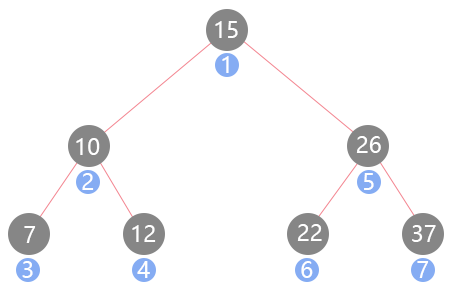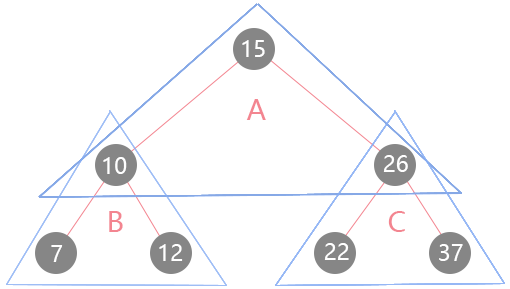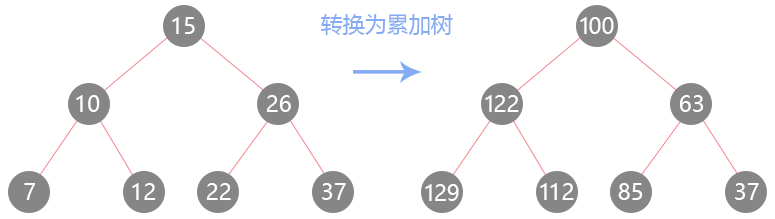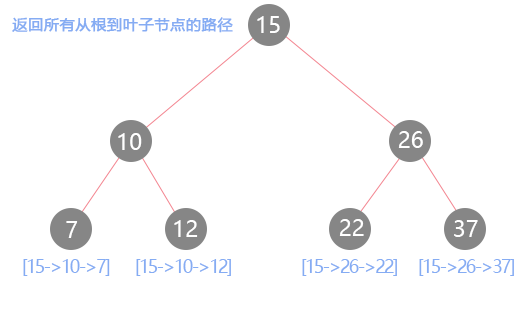九路103 0 0

### 深度优先遍历(DFS)

#### 前序遍历

class BST {
constructor() {
this.root = null // 根节点
}
...

preorder(fn) { // 前序遍历
const _helper = node => {
if (!node) {
return
}
fn(node.val) // 先访问根节点
_helper(node.left) // 再访问左节点
_helper(node.right) // 最后访问右节点
}
_helper(root)
}
}#### 前序遍历应用 - 108-将有序数组转换为二叉搜索树

将一个按照升序排列的有序数组，转换为一棵高度平衡二叉搜索树。

0
/ \
-3   9
/   /
-10  5 

function TreeNode(val) { // 树节点类
this.val = val;
this.left = this.right = null;
}

var sortedArrayToBST = function (nums) {
const _helper = (arr, l, r) => { // l为左边界、r为右边界
if (l > r) { // 递归终止条件
return null
}
const mid = l + (r - l) / 2 | 0 // 取数组中间值，并向下取整
const node = new TreeNode(arr[mid]) // 前序遍历，实例化为树节点
node.left = _helper(arr, l, mid - 1) // 将分割的左侧构建为二叉搜索树
node.right = _helper(arr, mid + 1, r) // 将分割的右侧构建为二叉搜索树
return node // 将构建好的树返回
}
return _helper(nums, 0, nums.length - 1) // 注意前闭后闭的区间方式
}; 

#### 中序遍历

class BST {
constructor() {
this.root = null // 根节点
}
...

inorder(fn) { // 中序遍历
const _helper = node => {
if (!node) {
return
}
_helper(node.left) // 先访问左孩子
fn(node.val) // 再访问根节点
_helper(node.right) // 最后访问右孩子
}
_helper(root)
}
} 

#### 中序遍历应用 - 530-二叉搜索树的最小绝对差

给你一棵所有节点为非负值的二叉搜索树，请你计算树中任意两节点的差的绝对值的最小值。 

var getMinimumDifference = function (root) {
let prev = null // 保存之前访问的节点
let minimum = Infinity // 取最大值
const _helper = node => {
if (!node) {
return
}
_helper(node.left)
if (prev !== null) { // 第一次访问没有prev节点
minimum = Math.min(minimum, node.val - prev.val) // 始终保存最小的值
}
prev = node // 将本次循环的节点缓存为上一个节点
_helper(node.right)
}
_helper(root)
return minimum // 返回最小差
}; 

#### 后序遍历

class BST {
constructor() {
this.root = null // 根节点
}
...

postorder(fn) { // 后序遍历
const _helper = node => {
if (!node) {
return
}
_helper(node.left) // 先访问左孩子
_helper(node.right) // 然后访问右孩子
fn(node.val) // 最后访问根节点
}
_helper(root)
}
} 

#### 后序遍历应用 - 563-二叉树的坡度

给定一个二叉树，计算整个树的坡度。

var findTilt = function (root) {
let tilt = 0
const _helper = (node) => {
if (!node) {
return 0 // 叶子节点的坡度为0
}
const l = _helper(node.left) // 先求出左子树的和
const r = _helper(node.right) // 再求出右子树的和
tilt += Math.abs(l - r) // 当前节点的坡度等于左右子树和的绝对差
return l + r + node.val // 返回子树和
}
_helper(root)
return tilt
}; 

### 深度优先遍历(DFS)应用进阶

#### 反常规中序遍历 - 538-把二叉搜索树转换为累加树

给定一个二叉搜索树，把它转换成为累加树，使得每个节点的值是原来的节点值加上所有大于它的节点值之和。var convertBST = function (root) {
let sum = 0
const _helper = (node) => {
if (!node) {
return null
}
_helper(node.right) // 先遍历右子树
node.val += sum // 右子树到底后逐个节点进行值的累加与覆盖
sum = node.val // 记录的就是上个被覆盖节点的值
_helper(node.left) // 最后遍历左子树
}
_helper(root)
return root // 返回新的累加树
}; 

#### 前序加后序遍历 - 257-二叉树的所有路径

给定一个二叉树，返回所有从根节点到叶子节点的路径。var binaryTreePaths = function (root) {
const ret = []
const _helper = (node, path) => {
if (!node) {
return
}
path.push(node.val) // 前序遍历访问，记录路径的每一步
_helper(node.left, path)
_helper(node.right, path)
if (!node.left && !node.right) { // 到达了叶子节点
ret.push(path.join('->')) // 将路径转换为字符串
}
path.pop() // 后序遍历访问，将访问过的路径节点弹出
}
_helper(root, [])
return ret
}; 

### 广度优先遍历(BFS)

#### 树的BFS实现

class BST {
constructor() {
this.root = null // 根节点
}
...

levelOrder(fn) {
if (!this.root) {
return
}
const queue = [this.root] // 首先入队根节点
while (queue.length > 0) {
const node = queue.shift() // 出队队首节点
fn(node.val) // 访问出队节点值
if (node.left) { // 入队左孩子
queue.push(node.left)
}
if (node.right) { // 入队右孩子
queue.push(node.right)
}
}
}
} 

#### 广度优先遍历应用 - 637-二叉树的层平均值

var averageOfLevels = function (root) {
if (root === null) {
return []
}
const queue = [root]
const ret = [] // 最终返回的结果
while (queue.length > 0) {
let len = queue.length
let sum = 0 // 记录每一层的总和
for (let i = 0; i < len; i++) { // 遍历每一层
const node = queue.shift()
sum += node.val
if (node.left) {
queue.push(node.left)
}
if (node.right) {
queue.push(node.right)
}
}
ret.push(sum / len) // 求出该层的平均
}
return ret
}; 

### 最后